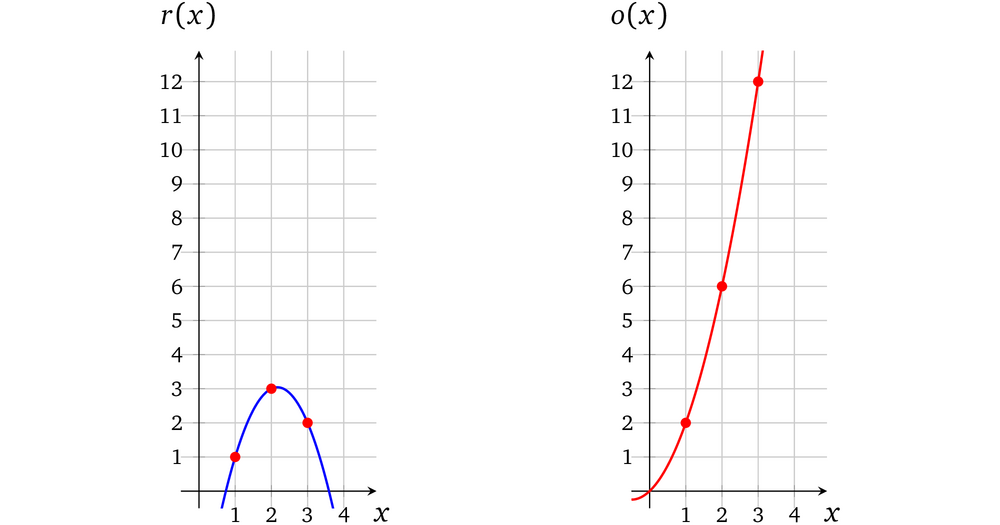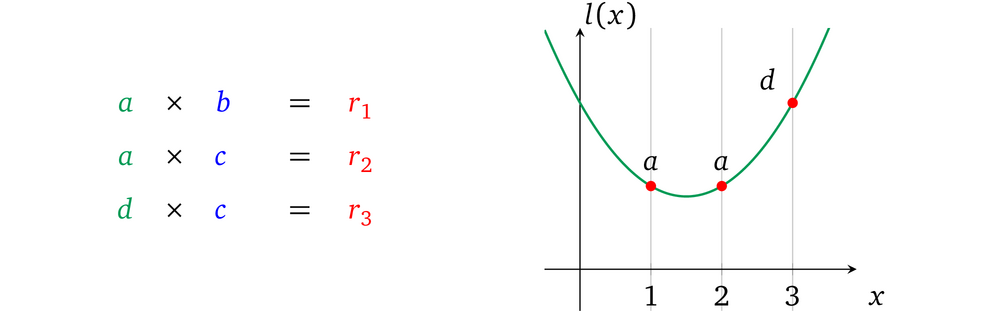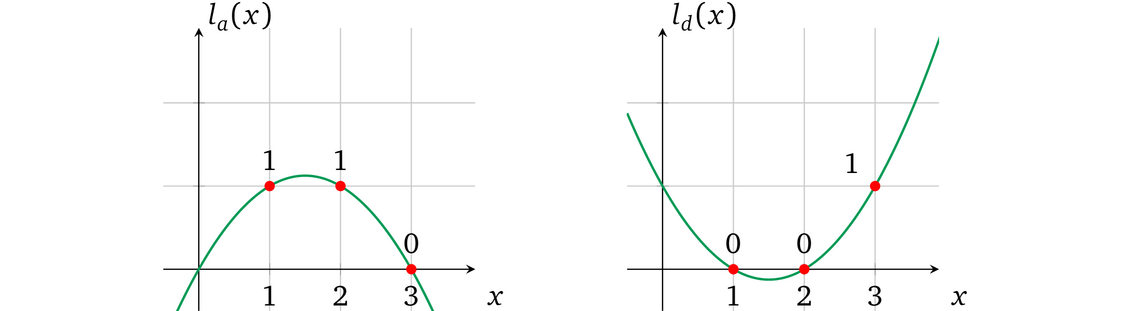### 从零开始学习zk-SNARK(三)—从程序到多项式的构造

even@安比实验室：前文主要介绍了如何构造多项式的零知识证明协议，现在将开始探讨如何构造更通用的协议。本节主要是讲如何将一组计算的证明转换为多项式进行证明。本文重点主要包括：多项式的算术性质，多项式插值等。

【本系列文章】

## 计算

Algorithm 1: Operation depends on an input
————————————————————————————————————————————————————————————————————

function calc(w, a, b)
if w then
return a × b
else
return a + b
end if
end function

f(w,a,b) = w(a · b)+(1-w)(a+b)​

w(a · b)+(1-w)(a+b) = 8​

even@安比实验室: 猜想一下，是否只要是能够用多项式表示的程序都可以做证明？

## 单个基本计算

$左操作数 \quad 运算操作符 \quad 右操作数 \quad = 输出$

### 多项式的算术性质

x = 1 时，计算 f(x) × g(x) 结果为：h(1) = – 8 + 66 – 193 + 231 – 90 = 6，也就是说当 x = 1时，h(x) 就是 f(x) 和 g(x) 相乘的结果 ，在 x 取其它值的时候也一样。

even@安比实验室: 回忆一下，在本系列的第一篇——多项式的性质与证明中，我们曾经说过“任何多项式在任意点的计算结果都可以看做是其唯一身份的表示。”

## 限制运算

l(x) 运算符 r(x) = o(x)​

• l(x) — 表示（运算结果为）左操作数
• r(x) — 表示（运算结果为）右操作数
• o(x) — 表示运算结果（输出）

l(x) × r(x) = o(x)

3x × 2x =6x

6x² -6x =0

6x² -6x = 6x(x-1)

$h(x)=\qquad\qquad\underline{\qquad\qquad 6x+2}$ $\ \ \ \ \ \ \ \ \ \ \ \ \ \ \ \ \ \ x-1) \quad 6x^2-4x \qquad$ $\ \ \ \ \ \ \ \ \ \ \ \ \ \ \ \ \ \ \ \ \ \ \ \ \ \ \ \ \ \ \underline{-6x^2+6x\qquad}$ $\ \ \ \ \ \ \ \ \ \ \ \ \ \ \ \ \ \ \ \ \ \ \ \ \ \ \ \ \ \ \ \ \ \ \ \ \ \ \ \ \ \ \ \ 2x \qquad$ $\ \ \ \ \ \ \ \ \ \ \ \ \ \ \ \ \ \ \ \ \ \ \ \ \ \ \ \ \ \ \ \ \ \ \ \ \ \ \ \ \ \underline{-2x+2}$ $\ \ \ \ \ \ \ \ \ \ \ \ \ \ \ \ \ \ \ \ \ \ \ \ \ \ \ \ \ \ \ \ \ \ \ \ \ \ \ \ \ \ \ \ \ \ \ \ \ \ \ \ \ 2$

$=> h(x)=6x+2+\frac{2}{x-1}$

even@安比实验室: 在前面的协议中，我们要证明的多项式是 p(x) = t(x)h(x)，这里我们修改 p(x)，使得 p(x) = l(x)r(x)-o(x)。这里t(x)目标多项式的根就是对应能够计算出数学表达式的值的 x。

## 运算的证明

e(gl(s), gr(s)) = e(gt(s),gh(s)) · e(go(s),g)

e(g,g)l(s)r(s) =e(g,g)t(s)h(s) ·e(g,g)o(s)

e(g,g)l(s)r(s) =e(g,g)t(s)h(s)+o(s)

• 证明

• 分配对应的系数给 $l(x)$，$r(x)$，$o(x)$
• 计算多项式 $h(x) = \frac{l(x) \times r(x)-o(x)}{t(x)}$
• 使用 ${g^{s^i}}_{i\in[d]}$ 计算加密多项式 $g^{l(s)},g^{r(s)},g^{o(s)}$ 和 $g^{h(s)}$
• 使用 ${g^{\alpha s^i}}_{i\in{0,…,d}}$ 计算加密多项式 $g^{αl(s)},g^{αr(s)},g^{αo(s)}$
• 设置证明 $π = (g^{l(s)},g^{r(s)},g^{o(s)},g^{h(s)},g^{αl(s)},g^{αr(s)},g^{αo(s)})$
• 验证

• 定义证明 $π$ 为 $(g^l,g^r,g^o,g^h,g^{l'},g^{r'},g^{o'})$

• 检查多项式约束

$e(g^{l'},g) = e(g^l,g^α)$

$e(g^{r'},g) = e(g^r,g^α)$

$e(g^{o'},g) = e(g^o,g^α)$

• 检查运算的有效性 $e(g^l,g^r) = e(g^{t(s)},g^h) \cdot e(g^o,g)$

even@安比实验室：上面例子里面取 x=1 这个特殊值作为运算编码的位置。当然这里的 1 可以换成任何别的值，比如说换成 x=2，3，或101 等等。在[GGPR]与[PHGR]论文中，这个取值是一个随机值，被称为 “root”。

## 多个运算

a × b = r1

r1 × c = r2

even@安比实验室：这里的示例为了理解方便，选择了 x=1, x=2 两个位置。记住在完整的 zkSNARK 方案中，这些 “root” 值必须为有限域内的随机数，否则就会引入安全漏洞。

2 × 1 = 2

2 × 3 = 6

6 × 2 = 12

### 多项式插值

• 一组未知方程
• 牛顿多项式
• 内维尔算法
• 拉格朗日多项式
• 快速傅里叶变换

$\begin{equation} \left{ \begin{array}{lr} l(1) = 2 \ l(2) = 2 \ l(3) = 6 \end{array} \right. => \left{ \begin{array}{lr} a(1)^2 +b \cdot 1 +c = 2 \ a(2)^2 +b \cdot 2 +c = 2 \ a(3)^2 +b \cdot 3 +c = 6 \end{array} \right. => \left{ \begin{array}{lr} a+b+c = 2 \ 4a+2b+c = 2 \ 9a+3b+c = 6 \end{array} \right. => \end{equation}$

$\begin{equation} \left{ \begin{array}{lr} a=2-b-c \ 2b=2-4(2-b-c)-c \ c=6-9(2-b-c)-3b \end{array} \right. => \left{ \begin{array}{lr} a=2-b-c \ b=\frac{6-3c}{2} \ c=-12+6b+9c \end{array} \right. => \end{equation}$

$\begin{equation} \left{ \begin{array}{lr} a=2-b-c \ 2b=\frac{6-3c}{2} \ c=-12+6(\frac{6-3c}{2})+9c \end{array} \right. => \left{ \begin{array}{lr} a=2 \ b=-6 \ c=6 \end{array} \right. \end{equation}$

l(x) = 2x2-6x+6

$\textcolor{blue}{r(x)}=\frac{-3x^2+13x-8}{2}; \quad \textcolor{red}{o(x)}=x^2+x$### 含有多个运算的多项式

$h(x) = \frac{l(x) \times r(x) -o(x)}{t(x)} = \frac{-3x^4 +22x^3 -57x^2 +63x -24}{(x-1)(x-2)(x-3)}$

\begin{align} h(x)=\qquad\qquad\qquad\qquad\underline{\qquad\qquad\qquad\qquad\qquad\qquad 3x+4} \ x^3-6x^2+11x-6)-3x^4+22x^3-57x^2+62x-24 \ \underline{\quad 3x^4-18x^3+33x^2-18x} \ {4x^3-24x^2+44x-24} \ \underline{\quad -4x^3+24x^2-44x+24} \ {0} \end{align}

even@安比实验室: 这里只需要一组多项式 l(x)，r(x)， o(x) 就可以将所有计算的约束关系表示出来了，有几个计算也就对应着目标多项式 t(x) 有几个根。

1）多个计算关系之间也是分开表示的，这些算式之间的关系也同样无法进行约束

2）由于 prover 生成的证明中只有计算结果，左操作数，右操作数，输出在计算中混用也不会被发现

3）由于左操作数，右操作数，输出是分开表示的，互相之间的关系无法进行约束

## 变量多项式

a × b = r1

a × c = r2

even@安比实验室：请务必注意“变量”的定义，它是程序中的变量，但是却又不能改，这不是很矛盾吗？其实，这里变量是指本文开头的示例伪代码中的那些不会被修改的变量。在 zkSNARK 论文中，这个「变量」其实有一个对应的名词叫做 assignment，是算术电路的「赋值」。而所有的assignments 是一个算术电路可满足性问题的解，包含了算术电路的输入值以及电路运算过程中输出的中间结果值。

### 单个变量的操作数多项式

2x2-6x+6=2×(x2 -3x+3)

• Setup
• 使用对应的系数构造相应的操作符多项式 l(x)
• 选择随机数 αs
• 使用加密的 l(s) 和它的”转换“：$(g^{l(s)},g^{αl(s)})$ 来设置 proving key
• 设置 verification key：$(g^α)$
• proving
• 对于操作数值 v
• 乘以操作数多项式：$(g^{l(s)})^v$
• 乘以变换后的操作数多项式：$(g^{αl(s)})^v$
• 提供 操作数多项式 的乘法证明： $(g^{vl(s)},g^{vαl(s)})$
• verification
• 解析证明为 $(g^l,g^{l'})$
• 验证比例：$e(g^{l'},g) = e(g^l,g^α)$

prover 需要返回同样的α-转换关系，因为无法从 proving key 中恢复出 α 所以保持这个变换的唯一方法就是用同一个值去分别乘以这两个加密值：gl(s)gαl(s)。这个 prover 就无法修改 l(x) 的单个系数了，例如如果多项式为 l(x) = ax2 + bx+ c ，prover 只可以用一个值 v 去修改整个多项式： v⋅(ax2 + bx+ c) = v⋅ax2 + v⋅bx+ v⋅c。配对 使其不能与另一个多项式做乘法，所以也就无法提供 s 的单个求幂值的 α-变换。 prover 不能做加法或者减法因为：

$g^{α(l(x)+a'x^2+c')} \not= g^{αl(x)} \cdot g^{a'x^2} \cdot g^{c'}$ 这里也同样需要未加密的 α 的知识。

Remark 4.1 由于 verification key 包含了加密了的 α，所以可以用多项式加（或者减）任意一个值 v'，即：

$g^{vl(s)} \cdot g^{v'} = g^{vl(s)+v'}$

$g^{αvl(s)} \cdot (g^α)^{v'} = g^{α(vl(s)+v')}$

$e(g^{α(vl(s)+v')},g) = e(g^{vl(s)+v'},g^α) 因而可以修改多项式使其超出 verifier 的预期以及证明一个不同的声明，后面的章节我们将会解决掉这个问题。 @even@安比实验室：这一小节是解决这样一个问题，算术电路中一个 input wire 或者 output wire可能同时会作为多个门的输入wire，如何确保约束这些公用wire的问题。 由于要证明的数学表达式是公开的，那么各个算式之间的约束关系也就是公开的，那么我们就可以把构造多项式的工作交给 setup 环节，这样 prover 只要填上对应的数值就可以了。 上文这个方法就限制了在同一个操作数多项式上，不同的计算式中使用的同一个值的约束关系；同样若一个操作数多项式中用到了多个值，也可以将这些值全都加起来，如下文所述。 ### 多变量的操作数多项式 因为只有当所有的左操作数使用同一个变量的时候我们才可以设置一个值。但是如果左操作数中再多一个值 d 要怎么做呢：假如我们还使用相同的方法，我们无法分别得为每一个变量设置值，并且让这些变量乘到一起去。也就是说这个受限的多项式只能支持一个变量的情况。我们可以把操作数多项式 l(x) 分成操作数的变量多项式$l_a(x)$和$l_d(x)$（注意下标） 这与上一节的方法一致，变量 ab 可以被赋值 并分别进行约束，然后加在一起就可以表示所有的左操作数变量了。因为我们将操作数的变量多项式 加在了一起，所以我们就要能够确保在每次计算中相加后的结果只表示所有操作符多项式 中的一个。 有了这个算术性质我们就可以逐一构造操作数变量多项式 了，这样如果变量 多项式在一个对应运算中被用做操作数，那么这一项就置为 1，否则就置为 0。0 跟任何值相乘结果都是零，当把他们相加在一起的时候也就可以忽略掉这一项。在我们的例子中这些变量多项式必须满足以下计算： la(1) =1, la(2)=1, la(3)=0 ld(x)=0, ld(2)=0, ld(3)=1 图中表示为：于是我们就可以将每个变量分开设置值，然后把他们加在一起来计算出操作数多项式，例如当 a = 3d= 2 时：注意：我们在一个值的后面使用下标表示它代表的变量，如, 3a 是一个用 3 实例化的变量 a。 现在起我们用大写字母来表示这个复杂的 操作符多项式，即 L(x) = ala(x) + dld(x) 计算结果为 L，也就是 L=L(s)。仅当每一个操作数的变量多项式 是由 verifier 约束的，结果才有效，与左多项式相关的交互应该相应得更改为： • Setup • 构造$l_a(x)$，$l_b(x)$使得它能够在对应的 “计算 x” 处为 1，在其他地方为 0。 • 选择随机数$s$，$α$• 计算并加密 未赋值的变量多项式：$g^{l_a(s)}$，$g^{l_d(s)}$• 计算变换后的这些多项式：$g^{αl_a(s)}$，$g^{αl_d(s)}$• 设置 proving key：$(g^{l_a(s)},g^{l_d(s)},g^{αl_a(s)},g^{αl_d(s)})$• 设置 verification key：$(g^α)$• Proving • 为变量多项式赋值 a 和 d：$（g^{l_a(s)}）^a$，$(g^{l_d(s)})^d$• 对变换后的变量多项式做同样的赋值：$(g^{αl_a(s)})^a$，$(g^{αl_d(s)})^d$• 把所有赋值好的变量多项式加起来变成操作数多项式的形式：$g^{L(s)}=g^{al_a(s)} \cdot g^{dl_d(s)} = g^{al_a(s)+dl_d(s)}$• 提供左操作数的有效证明：$(g^{L(s)},g^{αL(s)})$• Verification • 解析证明为$（g^L,g^{L’}）$• 验证提供的多项式是否是最初提供的多个未赋值的变量多项式 的和：$e(g^{L'},g) = e(g^L,g^α)$这里也就是验证了$αal_a(s) + αdl_d(s) = α \times (al_a(s) + dl_d(s))\$

• 除了“分配”值外，不能再修改它们的系数进而来修改变量多项式 了，因为 prover 只拿到了这些多项式的加密值，也因为 s 必要次幂的加密值不能与它们的 α 变换值一起使用
• 不能通过另一个多项式相加去提供一个结果因为这样 α-比例关系将会被破坏掉
• 不能通过与其他的一些多项式 u(x) 相乘来修改操作数多项式，这样可能会使得修改后的值不成比例因为在预配对空间中无法进行加密乘法

l'd(x) = cd·ld(x)+c'a·la(x)

L(x)=ca·la(x)+l'd(x) =(ca+c'a)·la(x)+cd·ld(x)

• 当 prover 决定不加入一些变量多项式 lᵢ(x) 来构造操作符多项式 L(x) 时依然是可以接受的，因为这和为它分配值为 0 是一样的：

gala(x) = gala(x)+0ld(x)

• 如果 prover 添加同一个变量多项式多次也是可以接受的因为这和一次分配多个值的和是一样的：

gala(x) · gala(x) · gala(x)= g3ala(x)

even@安比实验室: 总结一下本文证明协议的大致思路为：

1. 将要证明的程序转换为数学语言表达的形式（即加减乘除的计算）
2. 用多项式在某处的取值来进行计算以此表示数学计算，进而进行证明
3. 用多项式在多处的取值来进行计算表示多个数学运算，进而加以证明
4. 对证明的“程序”在不同计算中使用的相同的变量进行约束

https://arxiv.org/pdf/1906.07221.pdf

https://medium.com/@imolfar/why-and-how-zk-snark-works-4-general-purpose-computation-dcdc8081ee42

https://medium.com/@imolfar/why-and-how-zk-snark-works-5-variable-polynomials-3b4e06859e30

• 发表于 2020-01-30 17:50
• 阅读 ( 348 )
• 学分 ( 14 )
• 分类：零知识证明